Open In App
Related Articles
• Write an Interview Experience
• SQL for Data Science

# SQL | Join (Inner, Left, Right and Full Joins)

SQL Join statement is used to combine data or rows from two or more tables based on a common field between them. Different types of Joins are as follows:

• INNER JOIN
• LEFT JOIN
• RIGHT JOIN
• FULL JOIN
• NATURAL JOIN

Consider the two tables below as follows:

Student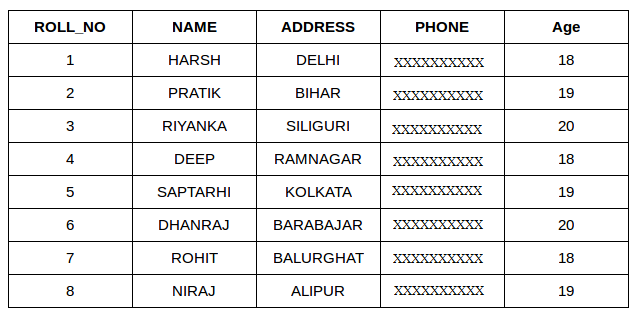StudentCourse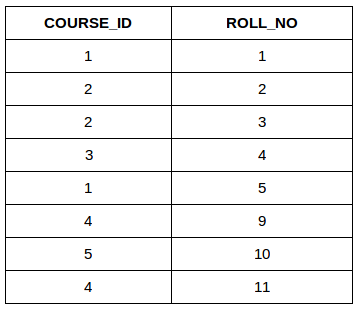The simplest Join is INNER JOIN.

### A. INNER JOIN

The INNER JOIN keyword selects all rows from both the tables as long as the condition is satisfied. This keyword will create the result-set by combining all rows from both the tables where the condition satisfies i.e value of the common field will be the same.

Syntax

```SELECT table1.column1,table1.column2,table2.column1,....
FROM table1
INNER JOIN table2
ON table1.matching_column = table2.matching_column;

table1: First table.
table2: Second table
matching_column: Column common to both the tables.```

Note: We can also write JOIN instead of INNER JOIN. JOIN is same as INNER JOIN.Example Queries(INNER JOIN)

This query will show the names and age of students enrolled in different courses.

```SELECT StudentCourse.COURSE_ID, Student.NAME, Student.AGE FROM Student
INNER JOIN StudentCourse
ON Student.ROLL_NO = StudentCourse.ROLL_NO;```

Output: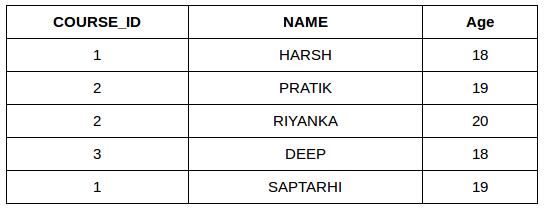### B. LEFT JOIN

This join returns all the rows of the table on the left side of the join and matches rows for the table on the right side of the join. For the rows for which there is no matching row on the right side, the result-set will contain null. LEFT JOIN is also known as LEFT OUTER JOIN.

Syntax:

```SELECT table1.column1,table1.column2,table2.column1,....
FROM table1
LEFT JOIN table2
ON table1.matching_column = table2.matching_column;

table1: First table.
table2: Second table
matching_column: Column common to both the tables.```

Note: We can also use LEFT OUTER JOIN instead of LEFT JOIN, both are the same.Example Queries(LEFT JOIN)

```SELECT Student.NAME,StudentCourse.COURSE_ID
FROM Student
LEFT JOIN StudentCourse
ON StudentCourse.ROLL_NO = Student.ROLL_NO;```

Output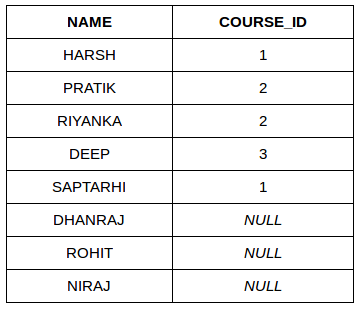### C. RIGHT JOIN

RIGHT JOIN is similar to LEFT JOIN. This join returns all the rows of the table on the right side of the join and matching rows for the table on the left side of the join. For the rows for which there is no matching row on the left side, the result-set will contain null. RIGHT JOIN is also known as RIGHT OUTER JOIN.

Syntax:

```SELECT table1.column1,table1.column2,table2.column1,....
FROM table1
RIGHT JOIN table2
ON table1.matching_column = table2.matching_column;

table1: First table.
table2: Second table
matching_column: Column common to both the tables.```

Note: We can also use RIGHT OUTER JOIN instead of RIGHT JOIN, both are the same.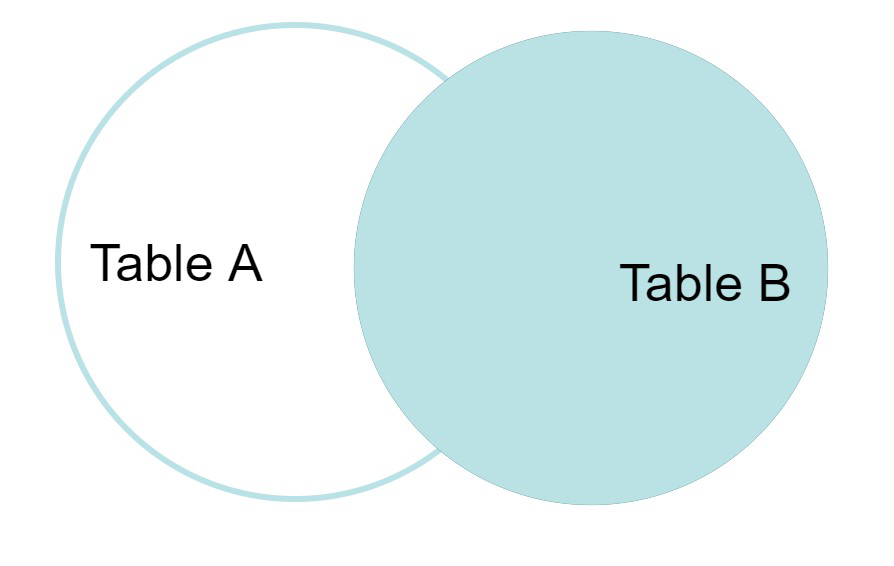Example Queries(RIGHT JOIN):

```SELECT Student.NAME,StudentCourse.COURSE_ID
FROM Student
RIGHT JOIN StudentCourse
ON StudentCourse.ROLL_NO = Student.ROLL_NO;```

Output: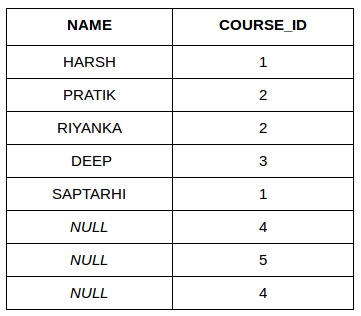### D. FULL JOIN

FULL JOIN creates the result-set by combining results of both LEFT JOIN and RIGHT JOIN. The result-set will contain all the rows from both tables. For the rows for which there is no matching, the result-set will contain NULL values.Syntax:

```SELECT table1.column1,table1.column2,table2.column1,....
FROM table1
FULL JOIN table2
ON table1.matching_column = table2.matching_column;

table1: First table.
table2: Second table
matching_column: Column common to both the tables. ```

Example Queries(FULL JOIN)

```SELECT Student.NAME,StudentCourse.COURSE_ID
FROM Student
FULL JOIN StudentCourse
ON StudentCourse.ROLL_NO = Student.ROLL_NO;```

Output:

### E. Natural join (⋈)

Natural join can join tables based on the common columns in the tables being joined. A natural join returns all rows by matching values in common columns having same name and data type of columns and that column should be present in both tables.

Both table must have at list one common column with same column name and same data type.

The two table are joined using Cross join.

DBMS will look for a common column with same name and data type Tuples having exactly same values in common columns are kept in result.

Example:

Query: Find all Employees and their respective departments.

Solution: (Employee) ⋈ (Department)

This article is contributed by Harsh Agarwal. If you like GeeksforGeeks and would like to contribute, you can also write an article using write.geeksforgeeks.org or mail your article to review-team@geeksforgeeks.org. See your article appearing on the GeeksforGeeks main page and help other Geeks.